In :
# %load ../../preconfig.py
%matplotlib inline
import matplotlib.pyplot as plt
import seaborn as sns
plt.rcParams['axes.grid'] = False

from __future__ import division

#import numpy as np
#import pandas as pd
#import itertools

import logging
logger = logging.getLogger()


# 19 Fibonacci Heaps¶

dual purpose:

1. support a set of operations of 'mergeable heap'.

2. its several Fibonacci-heap operations run in constant amortized time.

In :
plt.figure(figsize=(15,10))

Out:
<matplotlib.image.AxesImage at 0x10918be48>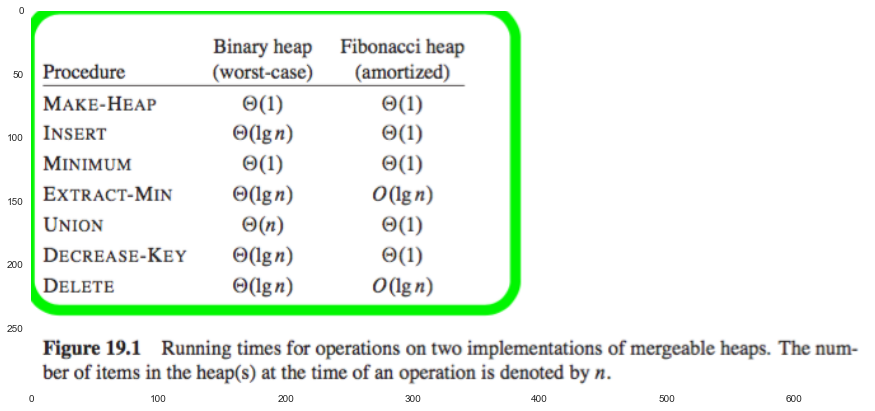In theory:
Fibonacci heaps are especially desiable when the number of EXTRAC-MIN and DELETE operations is small relative to the number of other operations performed.

In practice:
Ordinary binary heaps are more preferred because of the constant factors and programing complexity of Fibonacci heaps.

### 19.1 Structure of Fibonacci heaps¶

A Fibonacci heap is a collection of rooted trees that are min-heap ordered(the key of a node is greater than or equal to the key of its parent).

In :
plt.figure(figsize=(15,10))

Out:
<matplotlib.image.AxesImage at 0x10a47b5c0>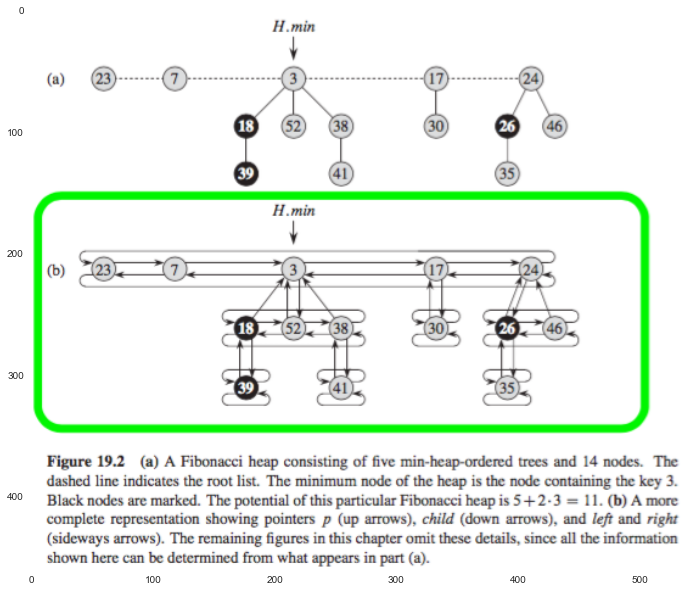1. each node $x$ contains a pointer $x.p$ to its parent and a pointer $x.child$ to any one of its children.

2. child list of $x$: The children of $x$ are linked together in a circular, doubly linked list. Each child $y$ in a child list has pointers $y.left$ and $y.right$.

• Insert or remove diretcly.
• Concatenate easily.

Attributes:

1. For each node $x$:

• $x.degree$: the number of children in the child list of node $x$.
• $x.mark$: whether node $x$ has lost a child since the last time $x$ was made the child of another node. (DECRESE-KEY operation).
2. For heap $H$:

• $H.min$: root pointer.
• $H.n$: the number of nodes currently in $H$.

#### Potential function¶

$$\phi (H) = t(H) + 2 m(H)$$

where $t(H)$ is the number of trees in the root list and $m(H)$ is the number of marked nodes.

#### Maximum degree¶

An upper bound $D(n)$ on the maximum degree of any node in an $n$-node Fibonacci heap: $D(n) \leq \lfloor \lg n \rfloor$.

### 19.2 Mergeable-heap operations¶

core: delay work as long as possible.

#### Creating a new Fibonacci heap¶

allocates and returns the Fibonacci heap object $H$, where $H.n = 0$ and $H.min = NIL$.

#### Inserting a node¶

insert $x$ into $H$'s root list

In :
plt.figure()
plt.figure()

Out:
<matplotlib.image.AxesImage at 0x10d283ba8>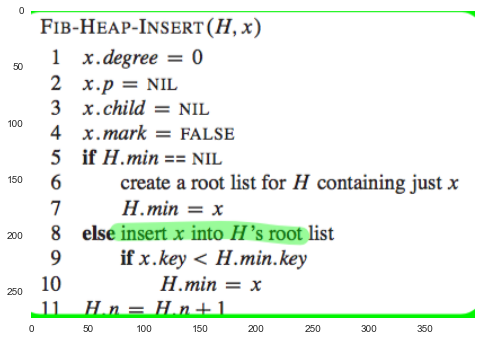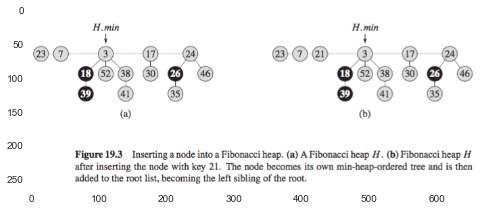#### Finding the minimum node¶

given by the pointer $H.min$

#### Uniting two Fibonacci heaps¶

concatenates the root lists of $H_1$ and $H_2$ and then determines the new minimum node.

In :
plt.imshow(plt.imread('./res/fig19_unit.png'))

Out:
<matplotlib.image.AxesImage at 0x10dd21630>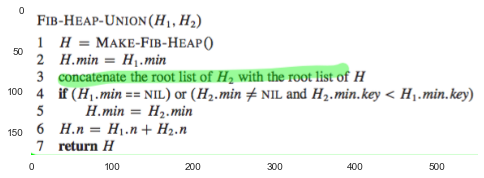#### Extracting the minimum node¶

$O(\lg n)$

extracte the minimum node, and also consolidate trees in the root list finally occurs.

In :
plt.imshow(plt.imread('./res/fig19_extract_min.png'))

Out:
<matplotlib.image.AxesImage at 0x10df49518>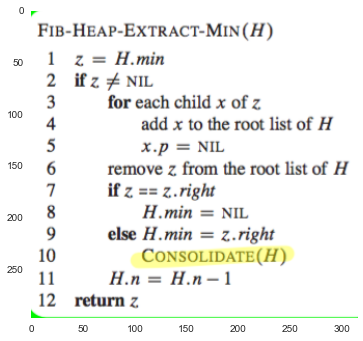##### consolidating¶

consolidating the root list consists of repeatedly excuting the following steps until every root in the root list has a distince degree values:

1. Find two roots $x$ and $y$ in the root list with the same degree. Let $x.key \leq y.key$.

2. Link $y$ to $x$: remove $y$ from the root list, and make $y$ a child of $x$ by calling the FIB-HEAP-LINK procedure.

In :
plt.figure(figsize=(20,15))
plt.subplot(2,1,1)

<matplotlib.image.AxesImage at 0x11b568588>